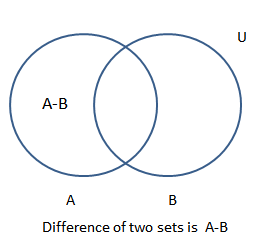### Difference of Two Sets CalculationThe difference of set B in set A is the complement of B in A.
If A and B are any two sets, then the relative complement
of B in A is the set of all elements in A which are not in B.
It is denoted by A - B. Difference of two sets A and B,
A - B is a set whose elements belong to A but not to B.
A - B is also called the relative complement of B

 Enter the elements of Universal set(A) seperated by comma Enter the elements of the set(B) seperated by comma Set Difference A - B